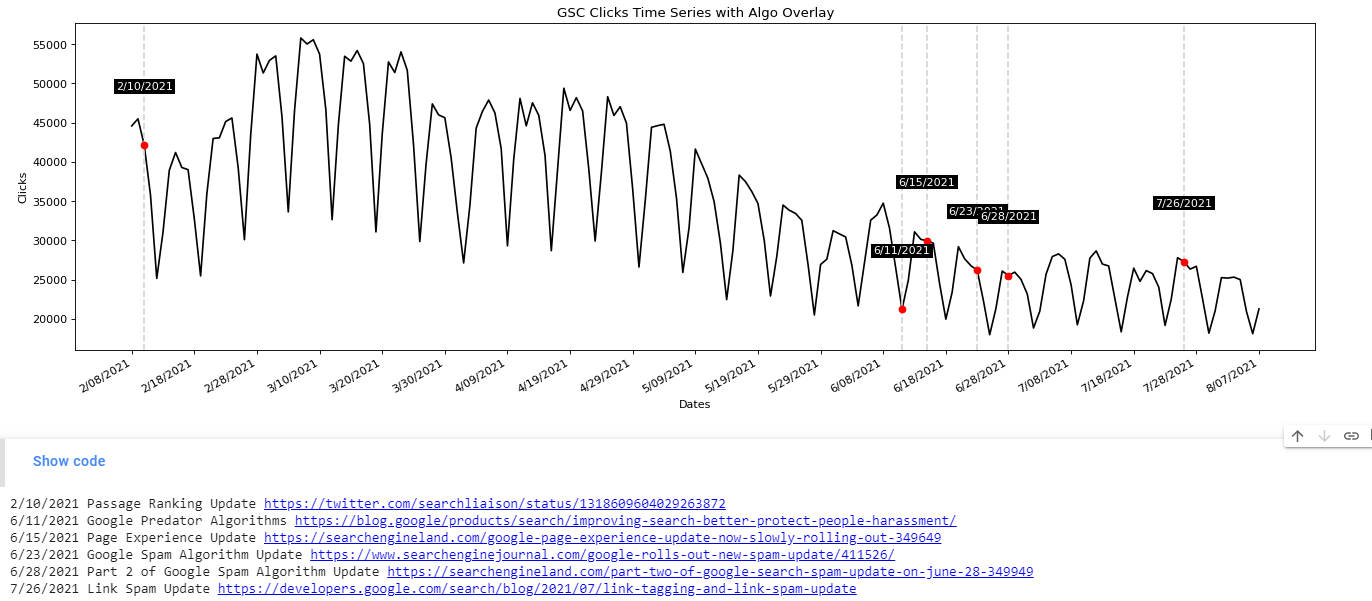Common Topics: data, algo, date, plt, gsc

Most SEO’s hearts skip a beat when they hear a Google algorithm update is unfolding and for a few days relentlessly check analytics. Then, there is a natural lull, the panic or excitement fades and you get back to your work. Google algorithms don’t always result in a dramatic spike one way or another. It can take weeks to determine a trend or a cause. In this tutorial, I’ll show you how to overlay your GSC data with known Google algorithm updates so you can better see a possible cause and effect.

The inspiration for this app and tutorial go to Signor Colt at iPullRank Agency for his most excellent data overlaying guide here. I wanted to try and put my own spin on this with this very specific application in mind.

Not interested in the tutorial? Head straight for the app here. The app gives you the ability to graph all 4 performance metrics.

Looking for a non-python professional option? Check out Seotesting’s Algorithm Update tool.

## Requirements and Assumptions

• Python 3 is installed and basic Python syntax understood
• GSC date performance data

## Import Python Modules

• json: for parsing the google algo list
• requests: to request the google algo list
• pandas: to import the GSC data
• matplotlib: for plotting/graphing
• figure: support for plotting/graphing
• datetime: to format date strings into date objects
• numpy: to help format the dates x-axis in the graphs

Let’s start the script off by importing those modules into the script.

```import json
import requests
import pandas as pd
import matplotlib.pyplot as plt
from matplotlib.pyplot import figure
from datetime import datetime
import numpy as np```

The first thing we’ll do is grab the algo list in JSON form available thanks to iPullRank. I am unsure if this list will be updated going forward. I may look to develop something if not. We then load the JSON data into updates_dict. I’m going to create 3 lists for each component of the algorithm (date, title, source), but I suppose you could create a 3 element list of lists. The data is then loaded into each list for use later on.

```updates = requests.get("https://ipullrank-dev.github.io/algo-worker/")

algo_notes = []
title = []

algo_notes.append(x['title'])
title.append(x['source'])```

Next, we import the GSC data. When exporting performance data you’ll be given a zip file. In the zip file you want the Dates.csv file. Then we need to convert the date from a string to a datetime object so we can next format it like the date in the Google Algo JSON data. Lastly, we sort the date values ascending so they are in oldest to newest for the graph.

```gsc = pd.read_csv("Dates.csv")
gsc['Date'] = gsc['Date'].astype('datetime64[ns]')
gsc['Date'] = gsc["Date"].dt.strftime('%-m/%d/%Y')
gsc = gsc.sort_values('Date',ascending=True)```

Now it’s time to start graphing! First, we set our axis data. GSC date will be the primary Y-Axis, GSC Clicks the primary X-Axis, and the Algo Dates a secondary Y-Axis. After setting some basics axis labels, we initialize the size of the graph with figure(). We use the plot() function to write the initial data plot points. The last parameter is color and line style. You can see a color guide here and a line type guide here. Lastly, we run the function autofmt_xdate() which automatically formats the date labels to fit the graph size.

```xs = gsc['Date']
ys = gsc['Clicks']

plt.title("GSC Clicks Time Series with Algo Overlay")
plt.xlabel("Dates")
plt.ylabel("Clicks")
figure(figsize=(20, 6), dpi=80)
plt.plot(xs,ys,'k-')
plt.gcf().autofmt_xdate()

algo_list = []```

The last graph part is to loop over each coordinate in the above initial graph and detect if a GSC date matches a date we have for a Google algo update.

If there is a match:

1.  Append the information to a new list where we will print out the details after graphing.
2.  Create a vertical grey dashed line at the position where the dates match
3.  Plot a red dot at the point where the dates match
4.  Create a text annotation at the point where they match that highlights the date where the algo matches a GSC date

Lastly, we handle pacing out the dates along the Y-Axis. If you have more than 30 days’ worth of data, the dates are going to be smashed together. We use the numpy function arrange() to calculate a range of dates from 0 to # of total dates uploaded, jumping by 10. We then shave off even more by altering the ticks to display every 10th date. This results in easy reading for a year or 6 months. If you are using 16 months I’d increase the jump number.

```for x,y in zip(xs,ys):

label = x

algo_list.append(x)
plt.axvline(x=x, color="lightgray", linestyle="--")
plt.plot(x,y,'ro')

plt.annotate(label,
(x,y),
color='white',
textcoords="offset points",
xytext=(0,50),
ha='center',

ind = np.arange(0, len(xs.index), 10)
plt.xticks(ind, xs[::10])
plt.show()```

All that is left to do now is print out the algorithm updates where those dates matched a date in the GSC data. We first create a loop through that list, record the index or numerical place for that update within the update list and then use it to grab the corresponding algo notes and titles as they all match in order.

```for x in algo_list: